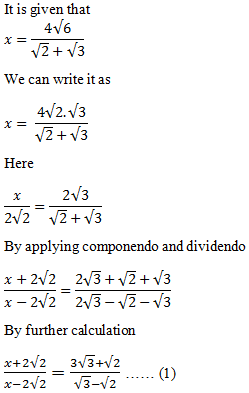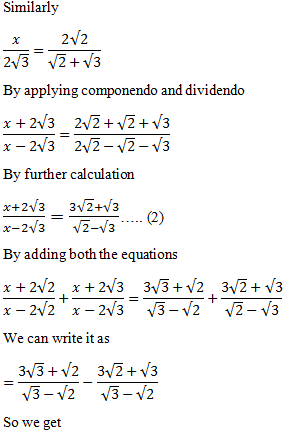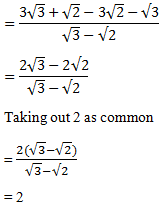Guru

# If x=4root6/root2+root3 find the value of (x+2root2)/(x-2root2) + (x+2root3)/(x-2root3)

• 0

This is a basic question from ML aggarwal book of class 10th, chapter 7, ratio and proportion., ICSE board

In this question we have been given that

x=4root6/(root2+root3)

And we have to find the value of (x+2root2)/(x-2root2) + (x+2root3)/(x-2root3)

Question 9, 7.3

Share